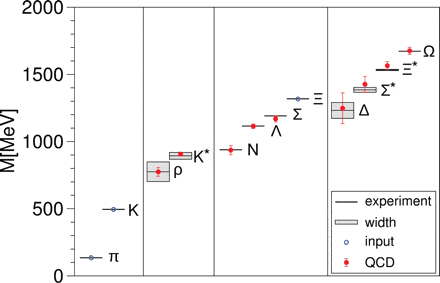# What measured values are used in the lattice QCD calculation of proton mass

kerrybbarnes
I've read that the mass of a proton has been calculated using QCD. But I assume some measured values are inputs to this calculation? If so can anyone provide a list of all of the values used (speed of light, Plank's constant, mass of other particles?)

humanino
Speed of light and Planck constants are mere definitions of units in this context, they are used indirectly (that is to say, chosen to be 1). Only enters mass of other particles, mostly pion, kaon and hyperon are used.

They usually take a few hadron masses as input to fix the scale. In hadron mass tables these fixed masses are always indicated.

kerrybbarnes
Thanks - that clears things up a bit. It could have been (for example) that Plank's mass was used to fix the scale. So saying that the mass of the proton has been calculated from first principles is a bit misleading? You could have said that the mass of a kaon was calculated and that the proton mass was used to fix the scale? Is a different simulation of each type of hadron done and then the mass ratios are compared? Why isn't it enough to choose the mass of just one particle to fix the scale? Is it just to improve the accuracy given the uncertainty of any one mass?

kerrybbarnes
Is more than one measured particle mass used because the exact mass of the quarks and gluons used is known even less well? Do these simulations reveal more about the mass of these particles?

I think two or three hadron masses are used. I have to check the references.

The calculation cannot be done based on first principles as even in the QCD Lagrangian neither masses nor the coupling constant is known. They are subject to renormalization ("running coupling constant"), so there are free parameters in the fundamental theory, not only for lattice gauge calculations.

kerrybbarnes
So if the masses and coupling constant you mentioned were known then the Lattice QCD method would determine the mass of the proton without any other masses? Suppose its two masses, ma and mb and one coupling constant, c. Then by running the GCD lattice mass determination for a range of these values you could determine a function for the mass of a proton Lp(ma,mb,c). If you did this for hadrons 1..n you could generate functions for each of these. Then by equating these functions to the measured values of the masses

m1 = L1(ma,mb,c)
m2 = L2(ma,mb,c)
...
mn = Ln(ma,mb,c)

You would have an overdetermined system of equations which would be a really great thing. Either they will have 0 solutions (indicating the QCD theory is wrong) 1 solution (in which case you'd have a unique determination of ma, mb and c, or an infinite number of solutions which would still mean you could use QCD to predict the value of a new hadron even though you don't know ma, mb and c directly.

I'm guessing this is all wrong but I'm trying to give you a target you can demolish. One of the things that I'm assuming is that a different Lattice QCD calculation was done for each type of hadron? Is even that part correct? Another assumption I'm making is that there are a finite number of free parameters - your comment - which I didn't understand - about "running coupling constants" makes me wonder if the situation isn't alot more complicated. But then how could two or three hadron masses fix the scale?

Last edited:
humanino
In addition to fixing the u,d and s masses, the choice of renormalization scale is also fixed by fitting whichever masses are chosen. It's a bit complicated to describe the entire story, but yes, you can count it as one single simulation. What is simulated once is a bunch of gluon configurations. Then some specific field operators have their expectation value calculated by a weighted sum over those configurations. This is essentially a brute force computation of the path integral (plus (many very) clever additional tricks).Ab-initio Determination of Light Hadron Masses

kerrybbarnes
Thanks humanino. The plot is a great help. Do I take it from what your saying ("you can count it as one single simulation") that a proton is never directly simulated? So my idea of individual hadrons being simulated is wrong and that there is some additional non-simulation transformation that takes the single simulation result and produces the mass of the different hadrons?

kerrybbarnes
Again - my naive assumption was that different hadrons like the proton were simulated using lattice QCD and the simulation revealed the mass of the hadron. I think from what has been said that this assumption is wrong? If so what would make it possible to actually simulate a proton directly? It seems like a desirable thing to do.

Last edited: